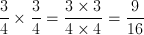# SSAT Upper Level Math : SSAT Upper Level Quantitative (Math)

## Example Questions

### Example Question #51 : Algebra

Micah is seeking new employment. The salary that he desires must be $4,000 per month with a tolerance of$600.  Which absolute value inequality can be used to assess the salary which will be tolerable?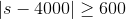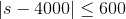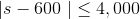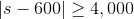Explanation:is the correct solution.

This absolute value inequality states that the difference between the salary of $4,000 must be less than or equal to$600 to be tolerable.

### Example Question #52 : Algebra

In order to ride the roller coaster at the local amusement park, children must be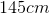tall with a tolerance of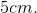Which of the following absolute value inequalities can be used to assess which heights will be tolerable?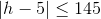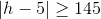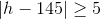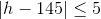Explanation:is the correct solution.

This Absolute Value inequality states that the difference between the children's height andmust be less than or equal to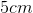.

### Example Question #53 : Algebra

Using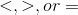compare the following absolute value.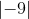__________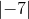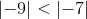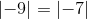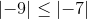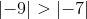Explanation:

The absolute value of a number is its distance from zero. The absolute value of a negative integer is a positive integer. The distance from  zero to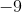on a number line is, so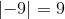The absolute value of a number is its distance from zero. The absolute value of a negative integer is a positive integer. The distance from 0 toon a number line     is. Therefore: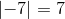Thereforebecause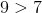### Example Question #12 : How To Find Absolute Value

Evaluate the expression ifand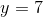.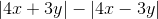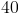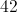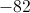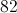Explanation:To solve, we replace each variable with the given value.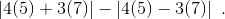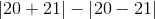Simplify. Remember that terms inside of the absolute value are always positive.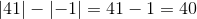### Example Question #26 : How To Find Absolute Value

Evaluate: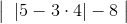Explanation: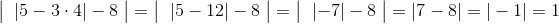### Example Question #1 : Generate Equivalent Numerical Expressions: Ccss.Math.Content.8.Ee.A.1

Evaluate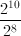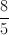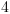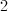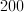Explanation:

If you divide two exponential expressions with the same base, you can simply subtract the exponents.  Here, both the top and the bottom have a base of 2 raised to a power.

So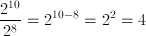### Example Question #1 : Generate Equivalent Numerical Expressions: Ccss.Math.Content.8.Ee.A.1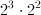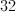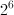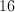Explanation:

Since the two expressions have the same base, we just add the exponents.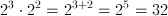### Example Question #11 : Generate Equivalent Numerical Expressions: Ccss.Math.Content.8.Ee.A.1

Evaluate: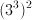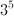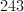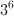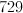Explanation:

A power raised to a power indicates that you multiply the two powers.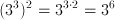### Example Question #1 : How To Find The Properties Of An Exponent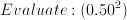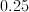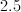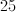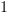Explanation:

We can either write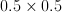, or we can convert this to a fraction and write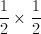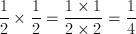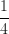in decimal form is 0.25.

### Example Question #51 : Ssat Upper Level Quantitative (Math)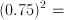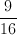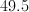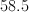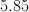Convert .75 to a fraction.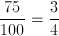.
Now multiply